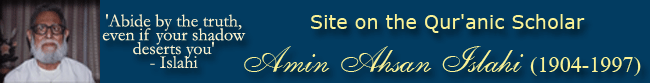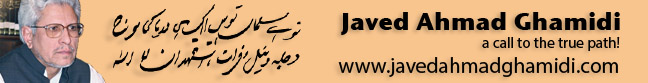Powered by UITechs

Post Reply
Username
Password
Format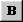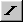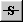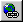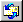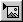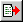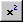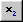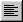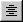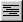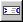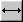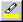Andale Mono Arial Arial Black Book Antiqua Century Gothic Comic Sans MS Courier New Georgia Impact Tahoma Times New Roman Trebuchet MS Script MT Bold Stencil Verdana Lucida Console 1 2 3 4 5 6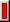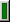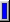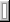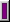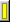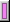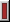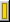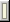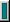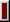Message Icon

Message

- Forum Code is ON
- HTML is OFF

 Smilies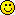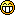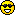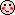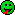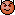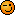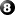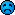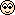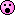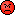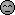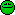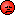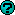Write in Urdu
اردو میں لکھيۓ
(For Urdu Forums Only)
Write here in Roman Urdu Preview

 A = آ a = ا
 B = N/A b = ب
 C = ث c = چ
 D = ڈ d = د
 E = N/A e = ع
 F = N/A f = ف
 G = غ g = گ
 H = ھ h = ح
 I = N/A i = ي
 J = ض j = ج
 K = خ k = ك
 L = N/A l = ل
 M = N/A m = م
 N = ں n = ن
 O = N/A o = ا
 P = N/A p = پ
 Q = N/A q = ق
 R = ڑ r = ر
 S = ص s = س
 T = ٹ t = ت
 U = ہ u = ء
 V = ظ v = ط
 W = N/A w = و
 X = ژ x = ش
 Y = N/A y = ے
 Z = ذ z = ز

Mode
Show Preview

 Copyright Studying-Islam © 2003-7  | Privacy Policy  | Code of Conduct  | An Affiliate of Al-Mawrid Institute of Islamic Sciences ® Top